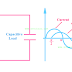Among the three types of electrical load according to nature or characteristics, Capacitive Load is one of them. Although there are very fewer applications of capacitive loads than others, but we have to know capacitive load examples, properties, and power consumption formulas. In fact, we must have knowledge about the properties of every electrical load, because we can arrange necessary systems and take precautions for that particular load.

The electrical load which is capacitive in nature and causes the consuming current to lead behind the voltage is called Capacitive Load. Like an inductive load capacitive load also consume both active and reactive power. The phase difference between current and voltage consumed by a pure capacitive load is 90 degrees leading. Although the practically pure capacitive load is not available. All capacitive loads are having capacitance as well as resistance properties.

Here some important examples of capacitive load are noted below,
• Synchronous Motor
• Capacitor Banks(although it can not be called an electrical load)
• TV Picture Tubes
• Buried Cables

## Capacitive Load Properties and Characteristics

1. Capacitive load consumes both active and reactive power.

2. A pure capacitive load has zero resistance although practically it is not possible, every capacitive load having some internal resistance.

3. The current consumed by a capacitive load always leads behind the voltage applied across the load.

4. A pure capacitive load causes the power factor zero leading but as there is no pure capacitive load so a capacitive load causes the power factor leading.

5. The capacitive load does not show the same effect for AC and DC, it provides high reactance to the DC current and low reactance to the AC current.

6. A capacitive load can store electrical energy in the form of electric charge and also can return back to the circuit or source.

7. Capacitive load are the very low power consuming loads compared to a resistive and inductive load.

8. Capacitive load does not waste the electrical energy like a resistive load.

## Power Consumed by Capacitive Load

The active power formula for a capacitive load is P = VIcosΦ, where P= power, V= voltage, I = current, and cosΦ = power factor

The reactive power formula for a capacitive load is  Q = VIsinΦ

1. Capacitive load such as synchronous motors are used for power factor improvement purpose as well as to drive mechanical loads also.

2. When capacitor banks are directly connected with a power supply without any other load then it does not consume any power means if it consumes power again returns back to the source, so a capacitor bank cannot be called an electrical load alone. When it is used with an inductive load parallelly then it consumes the reactive power to balance the power factor and then it can be called an electrical load which consumes reactive power only.

3. Other capacitive loads such as radio circuits, telecom circuits are used for electronic communication and signal transmission purposes.

Thank you for visiting the website. keep visiting for more updates.

Capacitive Load Examples, Properties, Power ConsumptionReviewed by Author on February 08, 2021 Rating: 5